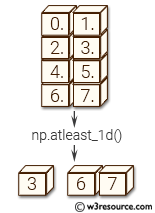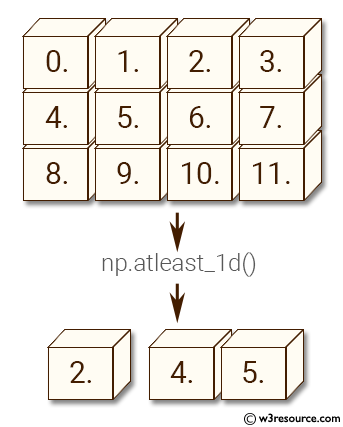# NumPy Array manipulation: atleast_1d() function

## numpy.atleast_1d() function

The numpy.atleast_1d() function is used to convert inputs to arrays with at least one dimension.
Scalar inputs are converted to 1-dimensional arrays, whilst higher-dimensional inputs are preserved.

Syntax:

`numpy.atleast_1d(*arys)`Version: 1.15.0

Parameter:

Name Description Required /
Optional
arys1, arys2, . . One or more input arrays. Required

Return value:

ret [ndarray] An array, or list of arrays, each with a.ndim >= 1. Copies are made only if necessary.

Example-1: numpy.atleast_1d()

``````>>> import numpy as np
>>> np.atleast_1d(2.0)
array([ 2.])
``````

Example-2: numpy.atleast_1d()

``````>>> import numpy as np
>>> a = np.arange(12.0).reshape(3,4)
>>> np.atleast_1d(a)
array([[  0.,   1.,   2.,   3.],
[  4.,   5.,   6.,   7.],
[  8.,   9.,  10.,  11.]])
``````

Pictorial Presentation:Example-3: numpy.atleast_1d()

``````>>> import numpy as np
>>> a = np.arange(12.0).reshape(3,4)
>>> np.atleast_1d(a) is a
True
>>> np.atleast_1d(2,[4, 5])
[array(), array([4, 5])]
``````

Python - NumPy Code Editor:

Previous: transpose()
Next: arleast_2d()

﻿